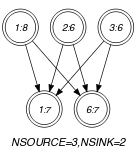## 5.76. common_interval

Origin

Derived from $\mathrm{𝚌𝚘𝚖𝚖𝚘𝚗}$.

Constraint

$\mathrm{𝚌𝚘𝚖𝚖𝚘𝚗}_\mathrm{𝚒𝚗𝚝𝚎𝚛𝚟𝚊𝚕}\left(\begin{array}{c}\mathrm{𝙽𝙲𝙾𝙼𝙼𝙾𝙽}\mathtt{1},\hfill \\ \mathrm{𝙽𝙲𝙾𝙼𝙼𝙾𝙽}\mathtt{2},\hfill \\ \mathrm{𝚅𝙰𝚁𝙸𝙰𝙱𝙻𝙴𝚂}\mathtt{1},\hfill \\ \mathrm{𝚅𝙰𝚁𝙸𝙰𝙱𝙻𝙴𝚂}\mathtt{2},\hfill \\ \mathrm{𝚂𝙸𝚉𝙴}_\mathrm{𝙸𝙽𝚃𝙴𝚁𝚅𝙰𝙻}\hfill \end{array}\right)$

Arguments
 $\mathrm{𝙽𝙲𝙾𝙼𝙼𝙾𝙽}\mathtt{1}$ $\mathrm{𝚍𝚟𝚊𝚛}$ $\mathrm{𝙽𝙲𝙾𝙼𝙼𝙾𝙽}\mathtt{2}$ $\mathrm{𝚍𝚟𝚊𝚛}$ $\mathrm{𝚅𝙰𝚁𝙸𝙰𝙱𝙻𝙴𝚂}\mathtt{1}$ $\mathrm{𝚌𝚘𝚕𝚕𝚎𝚌𝚝𝚒𝚘𝚗}\left(\mathrm{𝚟𝚊𝚛}-\mathrm{𝚍𝚟𝚊𝚛}\right)$ $\mathrm{𝚅𝙰𝚁𝙸𝙰𝙱𝙻𝙴𝚂}\mathtt{2}$ $\mathrm{𝚌𝚘𝚕𝚕𝚎𝚌𝚝𝚒𝚘𝚗}\left(\mathrm{𝚟𝚊𝚛}-\mathrm{𝚍𝚟𝚊𝚛}\right)$ $\mathrm{𝚂𝙸𝚉𝙴}_\mathrm{𝙸𝙽𝚃𝙴𝚁𝚅𝙰𝙻}$ $\mathrm{𝚒𝚗𝚝}$
Restrictions
 $\mathrm{𝙽𝙲𝙾𝙼𝙼𝙾𝙽}\mathtt{1}\ge 0$ $\mathrm{𝙽𝙲𝙾𝙼𝙼𝙾𝙽}\mathtt{1}\le |\mathrm{𝚅𝙰𝚁𝙸𝙰𝙱𝙻𝙴𝚂}\mathtt{1}|$ $\mathrm{𝙽𝙲𝙾𝙼𝙼𝙾𝙽}\mathtt{2}\ge 0$ $\mathrm{𝙽𝙲𝙾𝙼𝙼𝙾𝙽}\mathtt{2}\le |\mathrm{𝚅𝙰𝚁𝙸𝙰𝙱𝙻𝙴𝚂}\mathtt{2}|$ $\mathrm{𝚛𝚎𝚚𝚞𝚒𝚛𝚎𝚍}$$\left(\mathrm{𝚅𝙰𝚁𝙸𝙰𝙱𝙻𝙴𝚂}\mathtt{1},\mathrm{𝚟𝚊𝚛}\right)$ $\mathrm{𝚛𝚎𝚚𝚞𝚒𝚛𝚎𝚍}$$\left(\mathrm{𝚅𝙰𝚁𝙸𝙰𝙱𝙻𝙴𝚂}\mathtt{2},\mathrm{𝚟𝚊𝚛}\right)$ $\mathrm{𝚂𝙸𝚉𝙴}_\mathrm{𝙸𝙽𝚃𝙴𝚁𝚅𝙰𝙻}>0$
Purpose

$\mathrm{𝙽𝙲𝙾𝙼𝙼𝙾𝙽}\mathtt{1}$ is the number of variables of the collection of variables $\mathrm{𝚅𝙰𝚁𝙸𝙰𝙱𝙻𝙴𝚂}\mathtt{1}$ taking a value in one of the intervals derived from the values assigned to the variables of the collection $\mathrm{𝚅𝙰𝚁𝙸𝙰𝙱𝙻𝙴𝚂}\mathtt{2}$: To each value $v$ assigned to a variable of the collection $\mathrm{𝚅𝙰𝚁𝙸𝙰𝙱𝙻𝙴𝚂}\mathtt{2}$ we associate the interval $\left[\mathrm{𝚂𝙸𝚉𝙴}_\mathrm{𝙸𝙽𝚃𝙴𝚁𝚅𝙰𝙻}·⌊v/\mathrm{𝚂𝙸𝚉𝙴}_\mathrm{𝙸𝙽𝚃𝙴𝚁𝚅𝙰𝙻}⌋,\mathrm{𝚂𝙸𝚉𝙴}_\mathrm{𝙸𝙽𝚃𝙴𝚁𝚅𝙰𝙻}·⌊v/\mathrm{𝚂𝙸𝚉𝙴}_\mathrm{𝙸𝙽𝚃𝙴𝚁𝚅𝙰𝙻}⌋+\mathrm{𝚂𝙸𝚉𝙴}_\mathrm{𝙸𝙽𝚃𝙴𝚁𝚅𝙰𝙻}-1\right]$.

$\mathrm{𝙽𝙲𝙾𝙼𝙼𝙾𝙽}\mathtt{2}$ is the number of variables of the collection of variables $\mathrm{𝚅𝙰𝚁𝙸𝙰𝙱𝙻𝙴𝚂}\mathtt{2}$ taking a value in one of the intervals derived from the values assigned to the variables of the collection $\mathrm{𝚅𝙰𝚁𝙸𝙰𝙱𝙻𝙴𝚂}\mathtt{1}$: To each value $v$ assigned to a variable of the collection $\mathrm{𝚅𝙰𝚁𝙸𝙰𝙱𝙻𝙴𝚂}\mathtt{1}$ we associate the interval $\left[\mathrm{𝚂𝙸𝚉𝙴}_\mathrm{𝙸𝙽𝚃𝙴𝚁𝚅𝙰𝙻}·⌊v/\mathrm{𝚂𝙸𝚉𝙴}_\mathrm{𝙸𝙽𝚃𝙴𝚁𝚅𝙰𝙻}⌋,\mathrm{𝚂𝙸𝚉𝙴}_\mathrm{𝙸𝙽𝚃𝙴𝚁𝚅𝙰𝙻}·⌊v/\mathrm{𝚂𝙸𝚉𝙴}_\mathrm{𝙸𝙽𝚃𝙴𝚁𝚅𝙰𝙻}⌋+\mathrm{𝚂𝙸𝚉𝙴}_\mathrm{𝙸𝙽𝚃𝙴𝚁𝚅𝙰𝙻}-1\right]$.

Example
$\left(3,2,〈8,6,6,0〉,〈7,3,3,3,3,7〉,3\right)$

In the example, the last argument $\mathrm{𝚂𝙸𝚉𝙴}_\mathrm{𝙸𝙽𝚃𝙴𝚁𝚅𝙰𝙻}=3$ defines the following family of intervals $\left[3·k,3·k+2\right]$, where $k$ is an integer. As a consequence the items of collection $〈8,6,6,0〉$ respectively correspond to intervals $\left[6,8\right]$, $\left[6,8\right]$, $\left[6,8\right]$ and $\left[0,2\right]$. Similarly the items of collection $〈7,3,3,3,3,7〉$ respectively correspond to intervals $\left[6,8\right]$, $\left[3,5\right]$, $\left[3,5\right]$, $\left[3,5\right]$, $\left[3,5\right]$, $\left[6,8\right]$. The $\mathrm{𝚌𝚘𝚖𝚖𝚘𝚗}_\mathrm{𝚒𝚗𝚝𝚎𝚛𝚟𝚊𝚕}$ constraint holds since:

• Its first argument $\mathrm{𝙽𝙲𝙾𝙼𝙼𝙾𝙽}\mathtt{1}=3$ is the number of intervals associated with the items of collection $〈8,6,6,0〉$ that also correspond to intervals associated with $〈7,3,3,3,3,7〉$.

• Its second argument $\mathrm{𝙽𝙲𝙾𝙼𝙼𝙾𝙽}\mathtt{2}=2$ is the number of intervals associated with the items of collection $〈7,3,3,3,3,7〉$ that also correspond to intervals associated with $〈8,6,6,0〉$.

Typical
 $|\mathrm{𝚅𝙰𝚁𝙸𝙰𝙱𝙻𝙴𝚂}\mathtt{1}|>1$ $\mathrm{𝚛𝚊𝚗𝚐𝚎}$$\left(\mathrm{𝚅𝙰𝚁𝙸𝙰𝙱𝙻𝙴𝚂}\mathtt{1}.\mathrm{𝚟𝚊𝚛}\right)>1$ $|\mathrm{𝚅𝙰𝚁𝙸𝙰𝙱𝙻𝙴𝚂}\mathtt{2}|>1$ $\mathrm{𝚛𝚊𝚗𝚐𝚎}$$\left(\mathrm{𝚅𝙰𝚁𝙸𝙰𝙱𝙻𝙴𝚂}\mathtt{2}.\mathrm{𝚟𝚊𝚛}\right)>1$ $\mathrm{𝚂𝙸𝚉𝙴}_\mathrm{𝙸𝙽𝚃𝙴𝚁𝚅𝙰𝙻}>1$ $\mathrm{𝚂𝙸𝚉𝙴}_\mathrm{𝙸𝙽𝚃𝙴𝚁𝚅𝙰𝙻}<$$\mathrm{𝚛𝚊𝚗𝚐𝚎}$$\left(\mathrm{𝚅𝙰𝚁𝙸𝙰𝙱𝙻𝙴𝚂}\mathtt{1}.\mathrm{𝚟𝚊𝚛}\right)$ $\mathrm{𝚂𝙸𝚉𝙴}_\mathrm{𝙸𝙽𝚃𝙴𝚁𝚅𝙰𝙻}<$$\mathrm{𝚛𝚊𝚗𝚐𝚎}$$\left(\mathrm{𝚅𝙰𝚁𝙸𝙰𝙱𝙻𝙴𝚂}\mathtt{2}.\mathrm{𝚟𝚊𝚛}\right)$
Symmetries
• Arguments are permutable w.r.t. permutation $\left(\mathrm{𝙽𝙲𝙾𝙼𝙼𝙾𝙽}\mathtt{1},\mathrm{𝙽𝙲𝙾𝙼𝙼𝙾𝙽}\mathtt{2}\right)$ $\left(\mathrm{𝚅𝙰𝚁𝙸𝙰𝙱𝙻𝙴𝚂}\mathtt{1},\mathrm{𝚅𝙰𝚁𝙸𝙰𝙱𝙻𝙴𝚂}\mathtt{2}\right)$ $\left(\mathrm{𝚂𝙸𝚉𝙴}_\mathrm{𝙸𝙽𝚃𝙴𝚁𝚅𝙰𝙻}\right)$.

• Items of $\mathrm{𝚅𝙰𝚁𝙸𝙰𝙱𝙻𝙴𝚂}\mathtt{1}$ are permutable.

• Items of $\mathrm{𝚅𝙰𝚁𝙸𝙰𝙱𝙻𝙴𝚂}\mathtt{2}$ are permutable.

• An occurrence of a value of $\mathrm{𝚅𝙰𝚁𝙸𝙰𝙱𝙻𝙴𝚂}\mathtt{1}.\mathrm{𝚟𝚊𝚛}$ that belongs to the $k$-th interval, of size $\mathrm{𝚂𝙸𝚉𝙴}_\mathrm{𝙸𝙽𝚃𝙴𝚁𝚅𝙰𝙻}$, can be replaced by any other value of the same interval.

• An occurrence of a value of $\mathrm{𝚅𝙰𝚁𝙸𝙰𝙱𝙻𝙴𝚂}\mathtt{2}.\mathrm{𝚟𝚊𝚛}$ that belongs to the $k$-th interval, of size $\mathrm{𝚂𝙸𝚉𝙴}_\mathrm{𝙸𝙽𝚃𝙴𝚁𝚅𝙰𝙻}$, can be replaced by any other value of the same interval.

Arg. properties
• Functional dependency: $\mathrm{𝙽𝙲𝙾𝙼𝙼𝙾𝙽}\mathtt{1}$ determined by $\mathrm{𝚅𝙰𝚁𝙸𝙰𝙱𝙻𝙴𝚂}\mathtt{1}$, $\mathrm{𝚅𝙰𝚁𝙸𝙰𝙱𝙻𝙴𝚂}\mathtt{2}$ and $\mathrm{𝚂𝙸𝚉𝙴}_\mathrm{𝙸𝙽𝚃𝙴𝚁𝚅𝙰𝙻}$.

• Functional dependency: $\mathrm{𝙽𝙲𝙾𝙼𝙼𝙾𝙽}\mathtt{2}$ determined by $\mathrm{𝚅𝙰𝚁𝙸𝙰𝙱𝙻𝙴𝚂}\mathtt{1}$, $\mathrm{𝚅𝙰𝚁𝙸𝙰𝙱𝙻𝙴𝚂}\mathtt{2}$ and $\mathrm{𝚂𝙸𝚉𝙴}_\mathrm{𝙸𝙽𝚃𝙴𝚁𝚅𝙰𝙻}$.

specialisation: $\mathrm{𝚌𝚘𝚖𝚖𝚘𝚗}$ ($\mathrm{𝚟𝚊𝚛𝚒𝚊𝚋𝚕𝚎}/\mathrm{𝚌𝚘𝚗𝚜𝚝𝚊𝚗𝚝}$ replaced by $\mathrm{𝚟𝚊𝚛𝚒𝚊𝚋𝚕𝚎}$).

Keywords
Arc input(s)

$\mathrm{𝚅𝙰𝚁𝙸𝙰𝙱𝙻𝙴𝚂}\mathtt{1}$ $\mathrm{𝚅𝙰𝚁𝙸𝙰𝙱𝙻𝙴𝚂}\mathtt{2}$

Arc generator
$\mathrm{𝑃𝑅𝑂𝐷𝑈𝐶𝑇}$$↦\mathrm{𝚌𝚘𝚕𝚕𝚎𝚌𝚝𝚒𝚘𝚗}\left(\mathrm{𝚟𝚊𝚛𝚒𝚊𝚋𝚕𝚎𝚜}\mathtt{1},\mathrm{𝚟𝚊𝚛𝚒𝚊𝚋𝚕𝚎𝚜}\mathtt{2}\right)$

Arc arity
Arc constraint(s)
$\begin{array}{c}\mathrm{𝚟𝚊𝚛𝚒𝚊𝚋𝚕𝚎𝚜}\mathtt{1}.\mathrm{𝚟𝚊𝚛}/\mathrm{𝚂𝙸𝚉𝙴}_\mathrm{𝙸𝙽𝚃𝙴𝚁𝚅𝙰𝙻}=\hfill \\ \mathrm{𝚟𝚊𝚛𝚒𝚊𝚋𝚕𝚎𝚜}\mathtt{2}.\mathrm{𝚟𝚊𝚛}/\mathrm{𝚂𝙸𝚉𝙴}_\mathrm{𝙸𝙽𝚃𝙴𝚁𝚅𝙰𝙻}\hfill \end{array}$
Graph property(ies)
 $•$$\mathrm{𝐍𝐒𝐎𝐔𝐑𝐂𝐄}$$=\mathrm{𝙽𝙲𝙾𝙼𝙼𝙾𝙽}\mathtt{1}$ $•$$\mathrm{𝐍𝐒𝐈𝐍𝐊}$$=\mathrm{𝙽𝙲𝙾𝙼𝙼𝙾𝙽}\mathtt{2}$

Graph class
 $•$$\mathrm{𝙰𝙲𝚈𝙲𝙻𝙸𝙲}$ $•$$\mathrm{𝙱𝙸𝙿𝙰𝚁𝚃𝙸𝚃𝙴}$ $•$$\mathrm{𝙽𝙾}_\mathrm{𝙻𝙾𝙾𝙿}$

Graph model

Parts (A) and (B) of Figure 5.76.1 respectively show the initial and final graph associated with the Example slot. Since we use the $\mathrm{𝐍𝐒𝐎𝐔𝐑𝐂𝐄}$ and $\mathrm{𝐍𝐒𝐈𝐍𝐊}$ graph properties, the source and sink vertices of the final graph are stressed with a double circle. Since the graph has only 3 sources and 2 sinks the variables $\mathrm{𝙽𝙲𝙾𝙼𝙼𝙾𝙽}\mathtt{1}$ and $\mathrm{𝙽𝙲𝙾𝙼𝙼𝙾𝙽}\mathtt{2}$ are respectively equal to 3 and 2. Note that the vertices corresponding to the variables that take values 0 or 3 were removed from the final graph since there is no arc for which the associated arc constraint holds.

##### Figure 5.76.1. Initial and final graph of the $\mathrm{𝚌𝚘𝚖𝚖𝚘𝚗}_\mathrm{𝚒𝚗𝚝𝚎𝚛𝚟𝚊𝚕}$ constraint(a) (b)